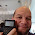## Saturday, December 15, 2012

### Trigonometric Functions with Random Number Arguments

Sine

Let R be a random number such that 0 ≤ R ≤ 1.

Then 0 ≤ R x ≤ x.

Since sin(0) = 0, we can simply conclude that 0 ≤ sin(R x) ≤ sin x, right?

However, we know that -1 ≤ sin x ≤ 1. Does this fact invalidate the claim that 0 ≤ sin(R x) ≤ sin x? Let's take a look at sin x vs sin(R x) graphically.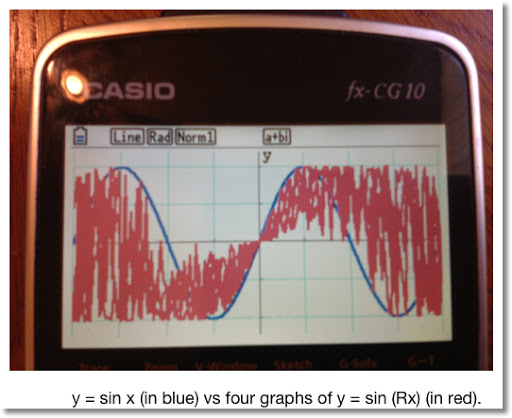From the graph, we can clearly see that 0 ≤ sin (Rx) ≤ sin x. Let's take a look at the region x ∈ [0, 2π].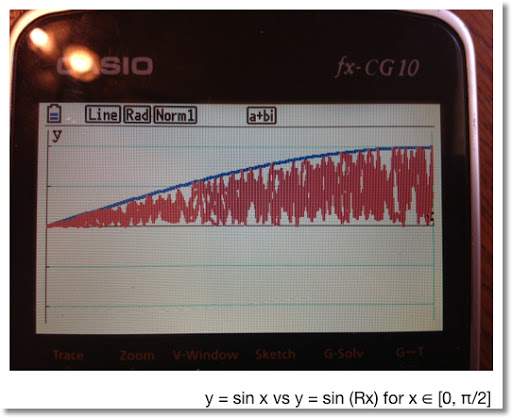In the region x ∈ [0, π/2], we can clearly see that 0 ≤ sin (Rx) ≤ sin x.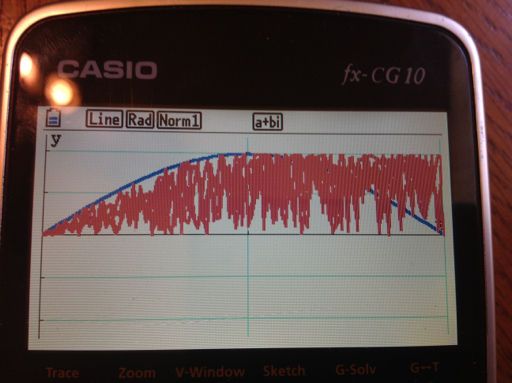As we explore the next region x ∈ [0, π], we still get positive values for sin (Rx). However, since R takes the value between 0 and 1, we get values 0 ≤ sin (Rx) ≤ 1.

Case: if R = 0.5 and x = π, sin(Rx) = 1.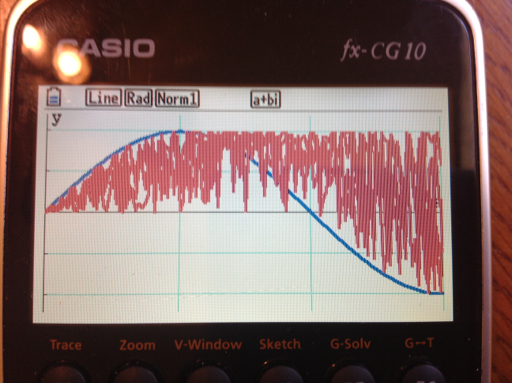When x ∈ [π/2, 3 π/2], the value of sin x declines below 0. However, since R is random, sin(Rx) takes the values sin x to 1.

Note that when x = 3π/2, sin(x) = -1.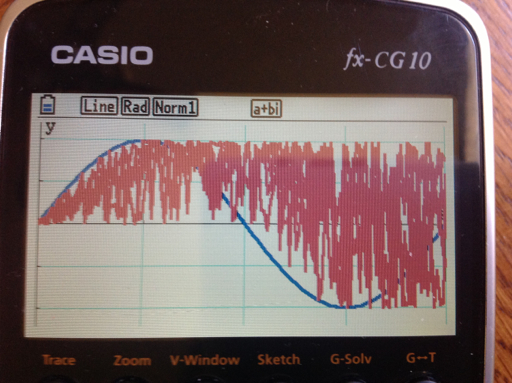At the final sub interval x ∈ [3π/2, 2 π], sin (Rx) takes on all values in the interval [-1, 1].

To summarize:

When 0 ≤ x ≤ π/2, 0 ≤ sin (Rx) ≤ sin x.
When π/2 ≤ x ≤ π, 0 ≤ sin (Rx) ≤ 1.
When π ≤ x ≤ 3π/2, sin x ≤ sin (Rx) ≤ 1.
When 3π/2 ≤ x ≤ 1, -1 ≤ sin (Rx) ≤ 1.

Interesting that we can't just say 0 ≤ sin (Rx) ≤ sin x for all x.

Cosine

We can make the similar argument that even though:

0 ≤ R ≤ 1, 0 ≤ R x ≤ x, and cos(0) = 1,

The statement 1 ≤ cos(Rx) ≤ cos x is not true for all x. Looking the graph cos x vs cos (Rx):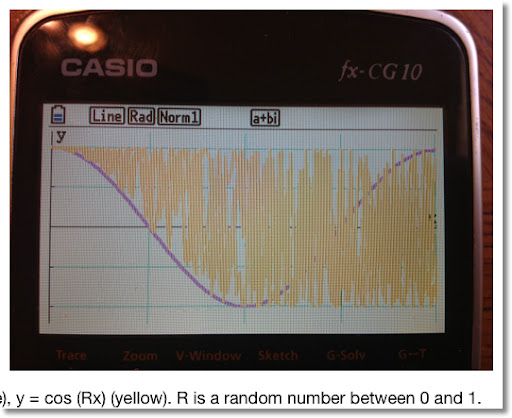Looking at the sub-intervals of [0, 2π]:

When 0 ≤ x ≤ π/2, cos x ≤ cos (Rx) ≤ 1.
When π/2 ≤ x ≤ π, π/2 ≤ cos (Rx) ≤ 1.
When π ≤ x ≤ 3π/2, -1 ≤ cos (Rx) ≤ 1.
When 3 π/2 ≤ x ≤ π, -1 ≤ cos (Rx) ≤ 1.

When random numbers are involved with functions, interesting things happen.

sin (Rx) cos (Rx) vs. 1/2 sin (2Rx)

Using the trig identity:

(sin x) * (cos x) = 1/2 * sin(2x)

Let R represent a random number function which returns a random number between 0 and 1. On a calculator, each time the random number function is called, a different random number is returned.

I will leave this as an open question: what happens at each interval [0, π/2], [π/2, π], [π, 3π/2], and [3π/2, 2 π]? I'll end this blog entry with a graphing comparison.

Note the range of sin(Rx) cos(Rx) is [-1, 1].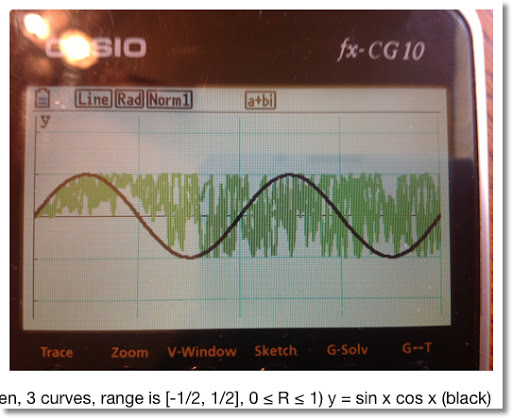Stay safe everyone! Eddie

This blog is property of Edward Shore. 2012

1.2.